##### Actions

(diff) ← Older revision | Latest revision (diff) | Newer revision → (diff)

A term of physical origin whose meaning in mathematics is not unambiguous. The adiabatic invariant is usually defined as a quantitative characteristic of the motion of a Hamiltonian system which remains practically unchanged during an adiabatic (i.e. very slow in comparison to the time scale typical of the motion in the system) change of its parameters. (The change may last for as long as the values of these parameters — unlike the adiabatic invariant — undergo significant changes.) Thus, for the simplest system(*)

whereis a small parameter and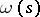is a positive, sufficiently smooth function, the adiabatic invariant isIf, the system (*) describes an ordinary harmonic oscillator with frequency. Thus, in this case, if the variation of the parameters of the system is slow in comparison to the oscillation period, then its energy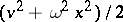varies proportionally to the frequency. In this example, as well as in most other cases, it is assumed that if the parameters did not change at all, the Hamiltonian system under consideration would be completely integrable and its motion would be quasi-periodic (in this case simply periodic); other generalizations are also known. In the publications by many mathematicians and experts in celestial mechanics on Hamiltonian systems which are close to being completely integrable, the term "adiabatic invariant" is not used at all, even though the information presented in these publications seems to indicate that certain magnitudes are in certain cases in fact adiabatic invariants.

"Approximate invariance" of an adiabatic invariant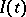means that for allunder consideration the difference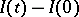remains small. (The derivative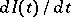can be a magnitude of the same order as the derivatives of the other parameters of the system, but the variations of the adiabatic invariant are not cummulative with time.) Such approximate invariance may take place over a very large but finite period of time (temporal adiabatic invariants) or over the entire infinite-axis (stationary or permanent adiabatic invariants ). The notion of "slow variation of the parameters of the system" may be rendered more precise in two ways: a) the Hamiltonian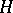is an explicit function of the time(non-autonomous system), but the derivative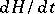is small; b) the system under consideration, with canonical variables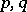, is a subsystem of a large system with variables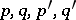, which is itself autonomous and is such that eithervary slowly, or else their variation does not materially affect the subsystem.

In view of the above restrictions, the existence of an adiabatic invariant can be affirmed only under various additional assumptions, a precise general formulation of which is difficult . Temporal adiabatic invariants for systems of the type just described in fact belong to the field of asymptotic methods of perturbation theory (if the problem is posed in a more general manner, a few rigorous results can also be obtained ). To prove the temporal asymptotic invariance of some magnitude, the usual procedure is to construct another magnitude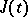such that the values ofoscillate around, whilehas a lower order of magnitude than the derivatives of the other parameters of the system. Thus, in the example (*) for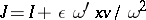(the prime denotes the derivative ofwith respect to the argument) direct differentiation yields; this is a proof thatis a temporal adiabatic invariant. The existence of permanent adiabatic invariants is open to doubt in case a) above ifdepends onin some general manner (in particular, in the absence of periodicity or limits as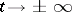). In case b) above, the problem of permanent adiabatic invariants is connected with small denominators .

Historically, adiabatic invariants played an important role in the quantum theory of Bohr–Sommerfeld, in which quantizable magnitudes must be adiabatic invariants. This rule lost its importance with the subsequent advances in quantum mechanics. Adiabatic invariants are used in modern physics in the study of motion of charged particles in electromagnetic fields . Here, the important magnitude is the ratio, whereis the magnetic field strength and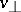is the velocity component of the particle lying in a plane perpendicular to the vector; this ratio is an adiabatic invariant if the magnetic field strength does not significantly vary along the Larmor radius.

It should also be noted that, during an adiabatic change of state in quantum mechanics, certain magnitudes retain their values (e.g. quantum numbers, except for processes which bring about a degenerate state of the system). It is therefore possible to speak of adiabatic invariants in quantum mechanics as well, but this concept does not play a significant part in quantum mechanics and is not ordinarily used in that science.

How to Cite This Entry: